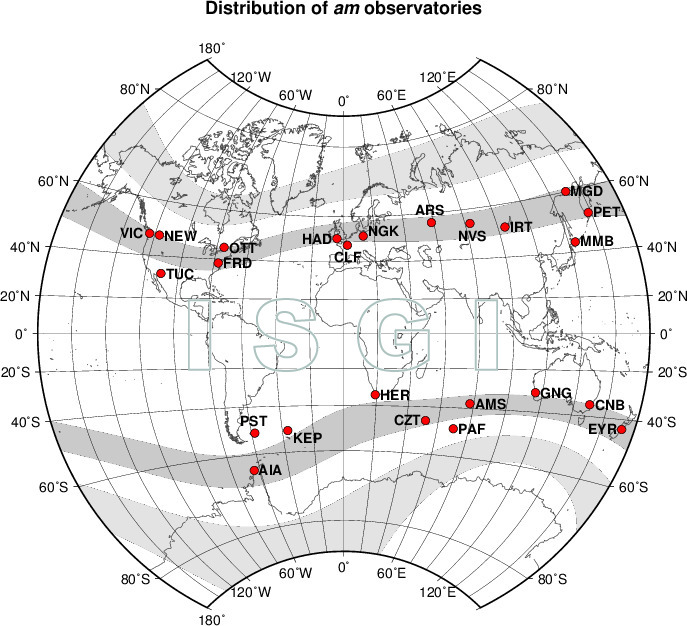Service International des Indices Géomagnétiques
International Service of Geomagnetic Indices

# am index

Index
 am time resolution: 3-hour (UT) intervalunit: nTAvailable from 1959 onwards

Type of index K-derived planetary (and hemispheric)

Purpose To provide a characterization of global geomagnetic activity using a large set of stations representing all longitudes and possible hemispheric discrepancies.

Network The stations are situated close to 50° corrected geomagnetic latitude (in the subauroral zones), and are gathered into groups, each corresponding to a longitude sector. There are 5 such groups in the Northern hemisphere, and 4 in the Southern one.

Derivation In each longitude sector, the K scaled for each station, are averaged, and the result is converted into amplitude using mid-class amplitudes for L9 = 500 nT (L9 being the K=9 lower limit; conversion table K2aK). The weighted average of the so-obtained amplitudes give rise to the hemispheric indices an (North) and as (South), the weighting factors accounting for the differences in the longitude sector extents in each hemisphere. The planetary index am is equal to (an + as) / 2.

An example of "Longitude Weighting Factors' computation principles" may be found here.

ISGI Collaborating Institute EOST, Strasbourg, France

IAGA endorsed IAGA Bulletin 27, 1969, p. 123, resolution 2

Related geomagnetic indices
License